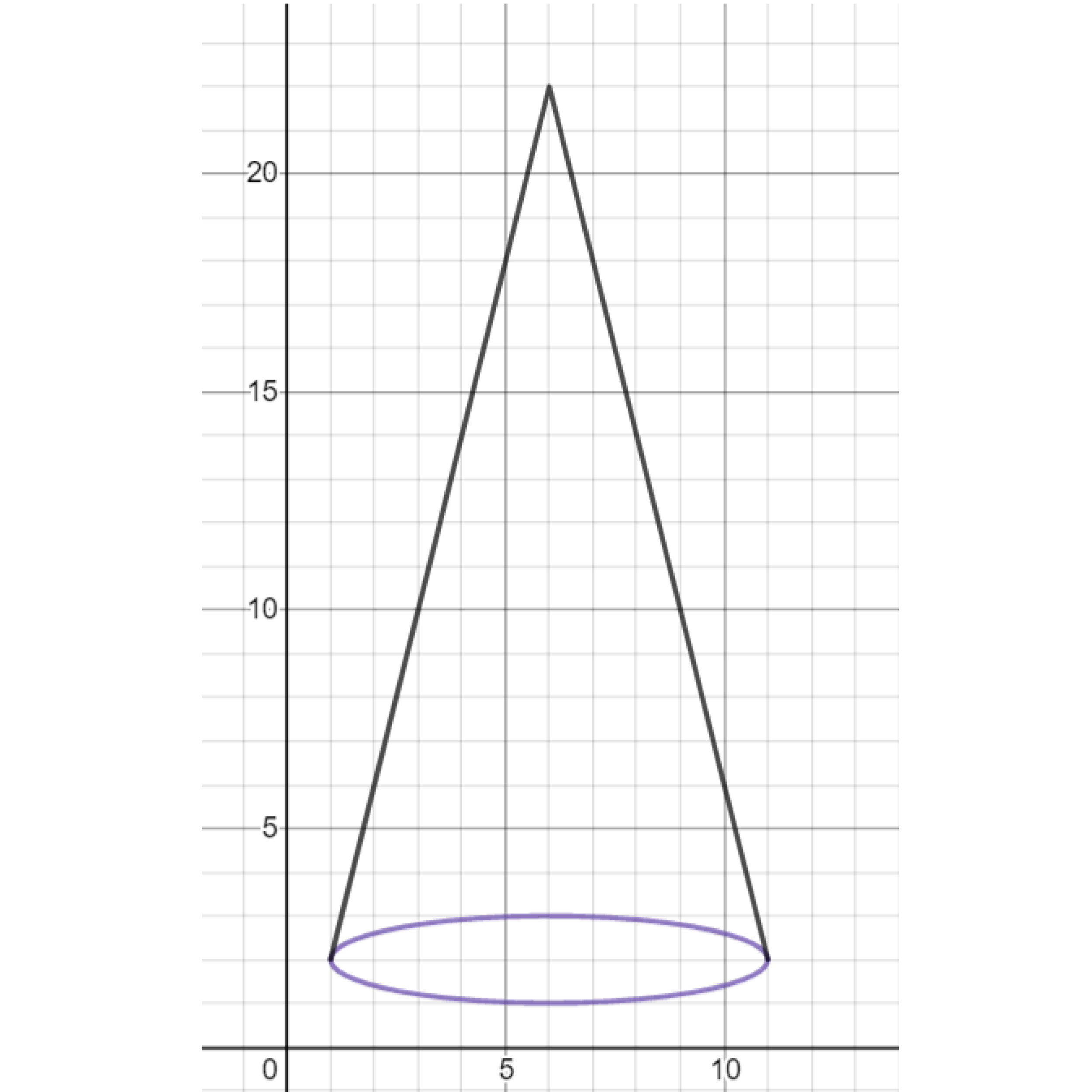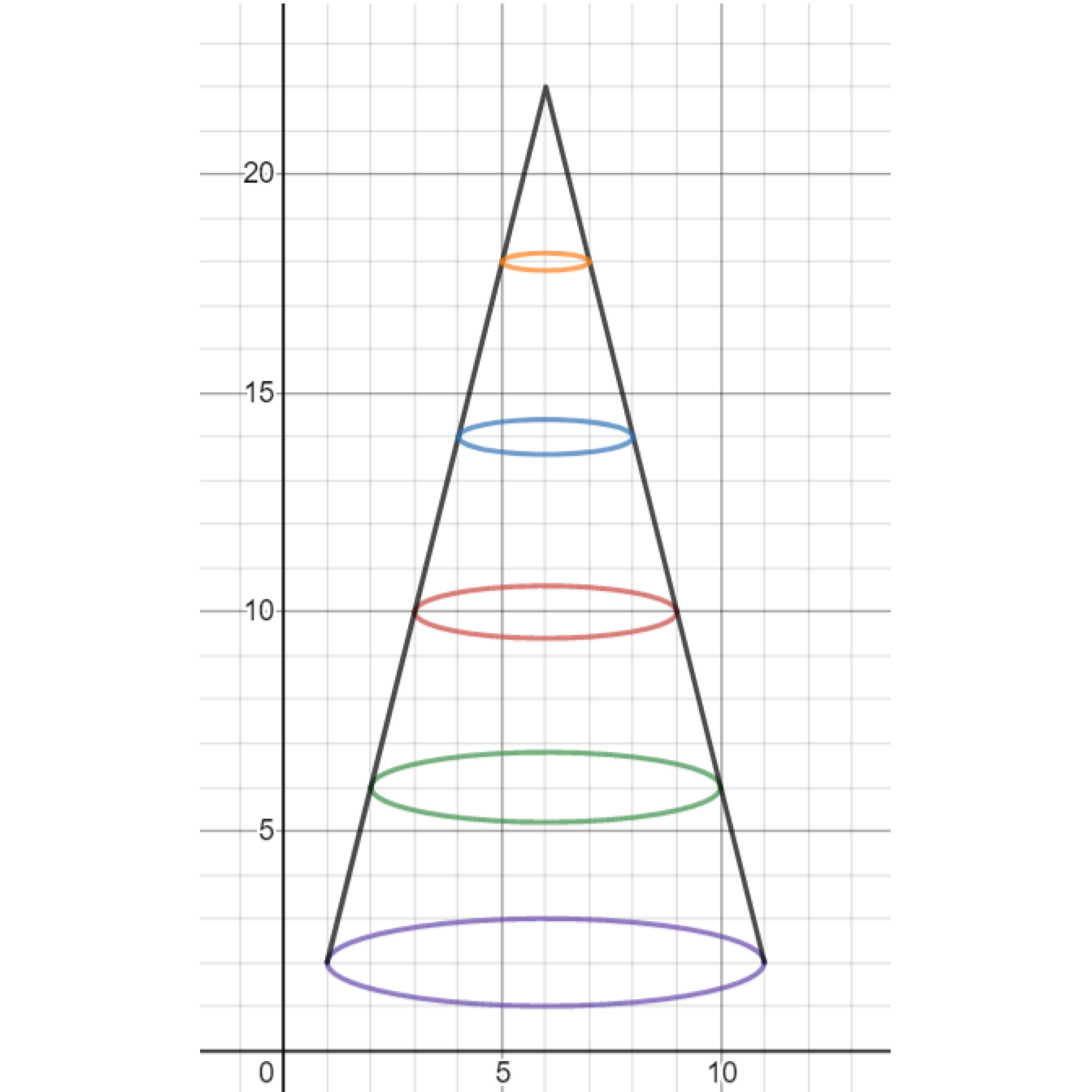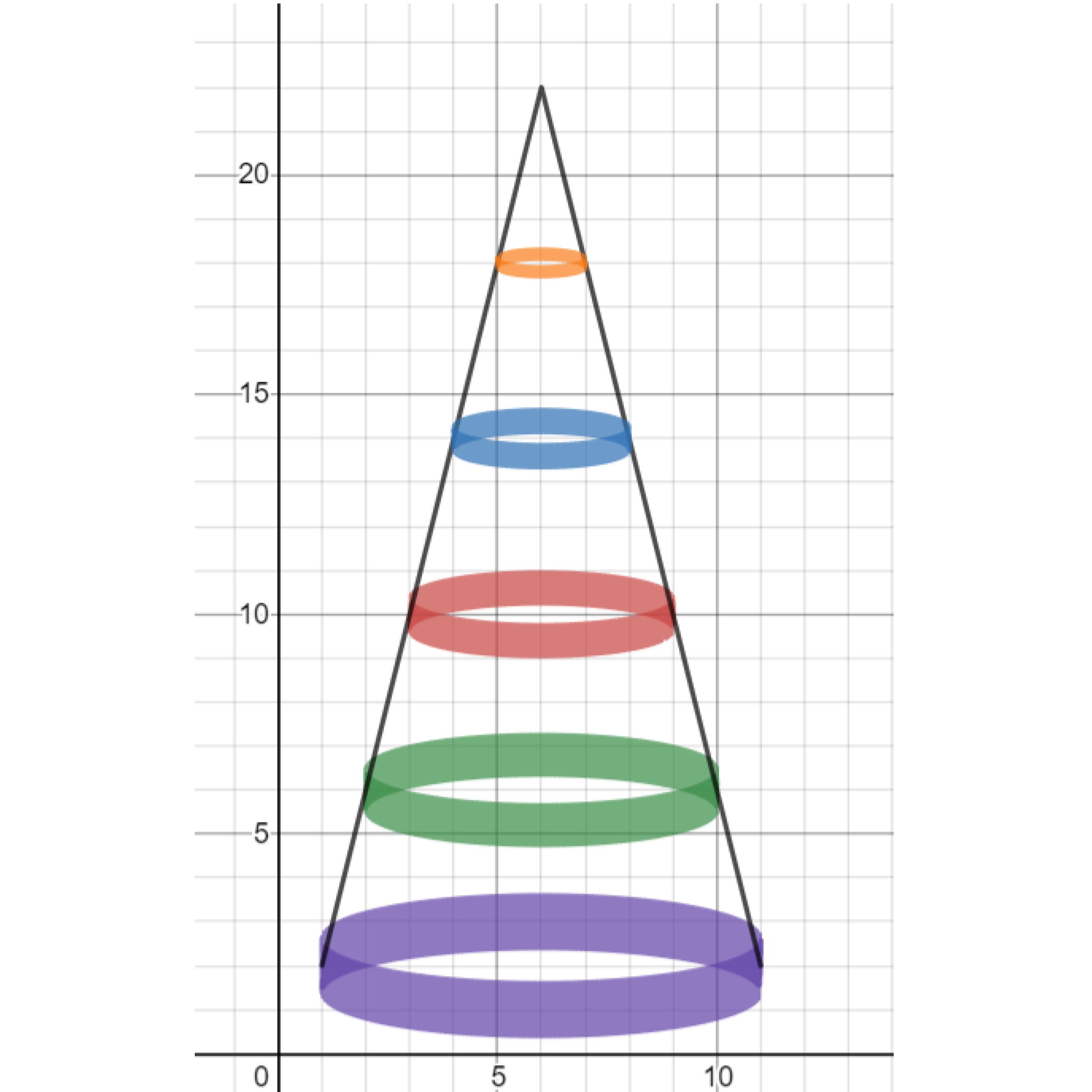# Graphing Calculator Drawing: Euclidean Ellipses

This post is part of a series.

Demonstration - Circles. Observe the graph as you type each of the following inputs. In general, the graph of $(x-a)^2+(y-b)^2=r^2$ makes a circle with radius $r$ centered at the point $(a,b).$

\begin{align*} &(x-0)^2+(y-0)^2=1^2 \\[10pt] &(x-2)^2+(y-3)^2 = 2^2 \\[10pt] &(x+5)^2+(y-2)^2=3^2 \end{align*}

Demonstration - Ellipses. Observe the graph as you type each of the following inputs. In general, the graph of $\left( \frac{x-a}{A} \right)^2 + \left( \frac{y-b}{B} \right)^2 = 1$ makes an ellipse with horizontal radius $A$ and vertical radius $B$ centered at the point $(a,b).$

\begin{align*} &\left( \frac{x-0}{1} \right)^2 + \left( \frac{y-0}{2} \right)^2 = 1 \\[10pt] &\left( \frac{x-2}{2} \right)^2 + \left( \frac{y-3}{5} \right)^2 = 1 \end{align*}

Demonstration - Shading. Observe the graph as you type each of the following inputs. In general, for large $v,$ the graph of $\left( \frac{x-a}{A} \right)^2 + \left( \frac{y+A\sin(vx)-b}{B} \right)^2 = 1$ shades vertically around the graph of $\left( \frac{x-a}{A} \right)^2 + \left( \frac{y-b}{B} \right)^2 = 1$ with thickness $2A.$

\begin{align*} &\left( \frac{x-0}{1} \right)^2 + \left( \frac{y+0.1\sin(1000x)-0}{2} \right)^2 = 1 \\[10pt] &\left( \frac{x-2}{2} \right)^2 + \left( \frac{y+0.4\sin(1000x)-3}{5} \right)^2 = 1 \end{align*}

Exercise. Use an absolute value function together with an ellipse to draw a cone.Exercise. Stack ellipses vertically on the cone.Exercise. Thicken the ellipses to form cylindrical shells.Challenge. Try stacking cylindrical shells on the peaks of $y=\sin x.$

This post is part of a series.

Tags: